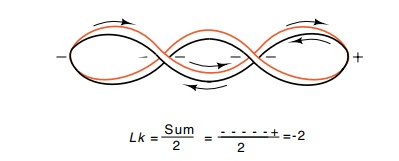Home | | Genetics and Molecular Biology | Determining Lk, Tw, and Wr in Hypothetical Structures

# Determining Lk, Tw, and Wr in Hypothetical Structures

A number of the fundamental processes in molecular biology involve the binding and interactions of proteins with DNA, and the covalent cutting and rejoining of DNA.

Determining Lk, Tw, and Wr in Hypothetical Structures

A number of the fundamental processes in molecular biology involve the binding and interactions of proteins with DNA, and the covalent cutting and rejoining of DNA. Much insight into these processes is provided by learning whether a portion of the DNA duplex is melted, and how the cutting and rejoining is performed. Often these processes affect the linking number or twist of the DNA, and both can be measured before and after the reaction. Then the effects of possible models on these numbers can be compared to experimental results. The determi-nation of linking number, twist, and superhelical turns in a structure can sometimes be tricky, and in the general situation is a relatively difficult mathematical problem. At our level of analysis, we can proceedFigure 2.12 Example of a structure with zero twist but a linking number of 2and hence containing two superhelical turns.

with straightforward approximations based on the fact that the linking number is the number of times that one DNA strand encircles the other. It is useful for this number to have a sign that is dependent on the orientation of the strands. Therefore, to determine the linking number of a structure, draw arrows on the two strands pointing in opposite directions, for example, pointing in the 5’ to 3’ direction on each. At each point where the two different strands cross, assign a + or - value dependent on the orientation. If the upper strand at a crossover can be brought into correspondence with the lower strand with a clockwiserotation, assign a +; if a counterclockwise rotation is required, assign a -. The linking number equals the sum of these values divided by 2, as shown in Fig. 2.12.

Viewing the DNA duplex as a ribbon provides one way to picture twist. Consider a straight line drawn perpendicular to the axis of the ribbon and through both edges. The rotation of this line as it is moved down the axis gives the twist. For a given structure, the writhing or superhelical turns is most easily given by whatever value Wr must have to make Lk = Tw + Wr.

Study Material, Lecturing Notes, Assignment, Reference, Wiki description explanation, brief detail
Genetics and Molecular Biology: Nucleic Acid and Chromosome Structure : Determining Lk, Tw, and Wr in Hypothetical Structures |